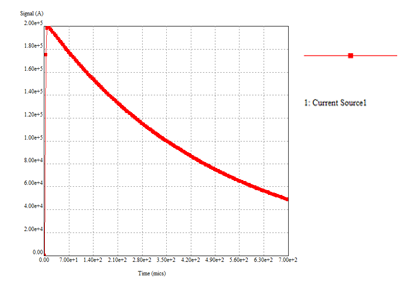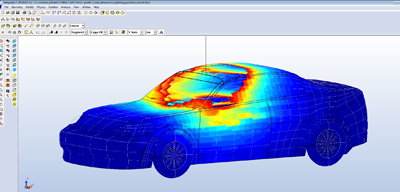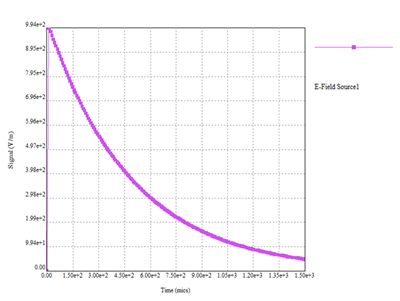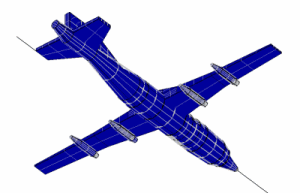APPLICATIONS

### Lightning Strikes Design

In the case of low frequency applications, the dimensions of the structure are very small compared to the wavelength. For example, a structure with dimensions 1m x 1m x 1m is subjected to an electromagnetic excitation of 30 kHz frequency. The wavelength (λ) corresponding to 30 kHz is 10km. Therefore the dimension of the structure in each direction is λ/10000.

Solving such problems through the FDTDconventional method requires hundreds of thousands or millions of time steps because the FDTD method is an explicit time-stepping technique with time steps limited by the Courant stability condition. Using very fine cell sizes causes high frequency errors which propagate within the FDTD grid. Consequently, as the time iterations increase, the numerical errors grow up and the solution becomes unstable.Another solver for this type of problem is the alternating direction implicit FDTD (ADI-FDTD). This unconditionally stable FDTD allows the use of a large time steps. A disadvantage of the ADI-FDTD is calculating matrix inversions, which places a heavy burden on the CPU time.

CHRONOS™ brings to the market a new approach to analyze a model. Thanks to the explicit FDTD Method, the software program tackles the instability problem by eliminating the high frequency errors at each time step. CHRONOS™ provides stable results for low frequency problems over millions of time step iterations, by suppressing the high frequency noise.

CHRONOS™ has the ability to solve high frequency and low frequency electromagnetic problems.

### Modeling of a Lightning Strike to a Car

Electromagnetic modeling of a car with dimensions of 4.8m x 1.75m x 1.3m as solved by CHRONOS™.

This problem is solved using 4,600,000 time steps for the lightning current, shown in Figure 1. The H-field is animated on the surface of the car as shown in Figure 2.### Modeling of a Lightning Strike to an Airplane

Electromagnetic modeling of an airplane with dimensions of 40m x 37m x 4m as solved by CHRONOS™.

This problem is solved using 1,737,000 time steps in order to simulate the electromagnetic interaction for a duration of 1650 μs. The lightning pulse waveform is shown in Figure 3. The E-field is animated on the surface of the airplane as shown in Figure 4.